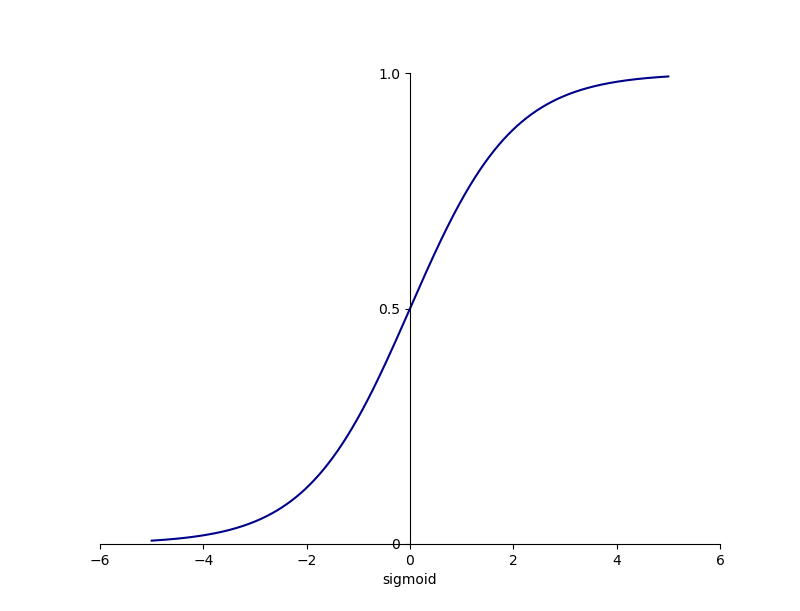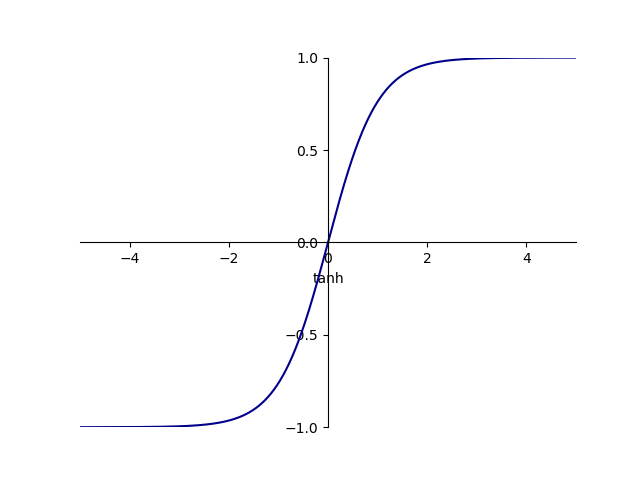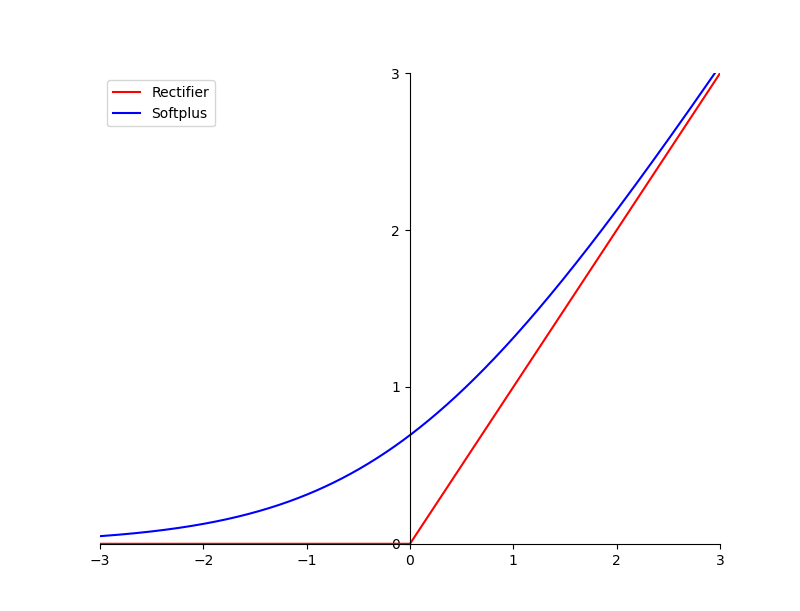## Sigmoid 函数

Sigmoid 激活函数的公式为：

Sigmoid(x)=\frac{1}{1+e^{-x}}

Sigmoid 函数可以将一个实数映射到 (0, 1) 范围内，可以用在 LSTM（Long Short-Term Memory，长短期记忆）中的各种门上，模拟门的关闭和开启状态。此外，Sigmoid 函数可以用来表示概率，还可以用于输入的归一化处理。Sigmoid 函数的图像如下所示：## Tanh 函数

Tanh 激活函数也是常见的激活函数，它可以将一个实数映射到 (-1, 1) 之间，其示意图如下所示：Tanh 函数的取值可以是负值，在某些需要抑制神经元的场景时，需要利用到该特性。例如，在 LSTM 中，可以利用 Tanh 函数的负值区来模拟“遗忘”。

Tanh 函数的公式为：

Tanh(x)=\frac{sinh(x)}{cosh(x)}=\frac{e^x-e^{-x}}{e^x+e^{-x}}=2Sigmoid(2x)-1

## ReLU 函数

f(x)=max(x, 0)

f(x)=ln(1+e^x)

Softplus 函数是对 ReLU 函数平滑逼近的解析形式，且它的导数恰好为 Sigmoid 函数。标准 ReLU 函数和 Softplus 函数的图像如下所示：• 单侧抑制。如上图所示，当输入值小于 0 时，神经元处于抑制状态；反之，神经元处于激活状态。而且 ReLU 函数求导比较简单，所以使用 ReLU 函数时随机梯度递减的收敛速度比 Sigmoid 和 Tanh 函数要快很多。
• 相对宽阔的兴奋边界。ReLU 函数在激活状态下的取值范围（当输入值大于 0 时的输出值范围）比 Sigmoid 和 Tanh 函数大得多。
• 稀疏激活。Sigmoid 函数将处于抑制状态的神经元设置了一个非常小的值，会增大计算负担。但 ReLU 函数将处于抑制状态的神经元的值直接设为 0，使得这些神经元不再参与后续的运算，增加了网络的稀疏性。